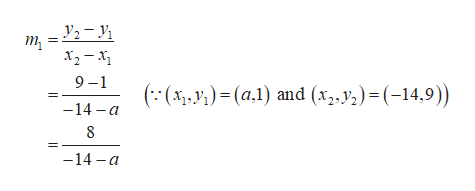If the line passing through the points(a, 1) and (−14, 9)is parallel to the line passing through the points(−2, 7) and (a + 2, 1),what is the value of a?

Question

If the line passing through the points

(a, 1) and (−14, 9)

is parallel to the line passing through the points

(−2, 7) and (a + 2, 1),

what is the value of a?

Step 1

The given points are

Step 2

Obtain the slope as follows.help_outlineImage Transcriptionclosey2-1 9 -1 (:()(a)and (*,))=(-14,9) -14 a 8 -14 a fullscreen
Step 3

The given poin...

Want to see the full answer?

See Solution

Want to see this answer and more?

Our solutions are written by experts, many with advanced degrees, and available 24/7

See Solution
Tagged in

Other